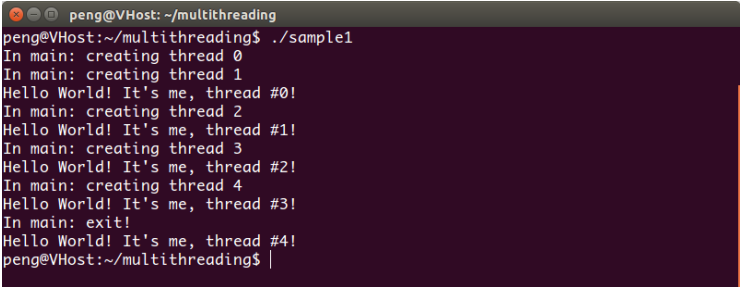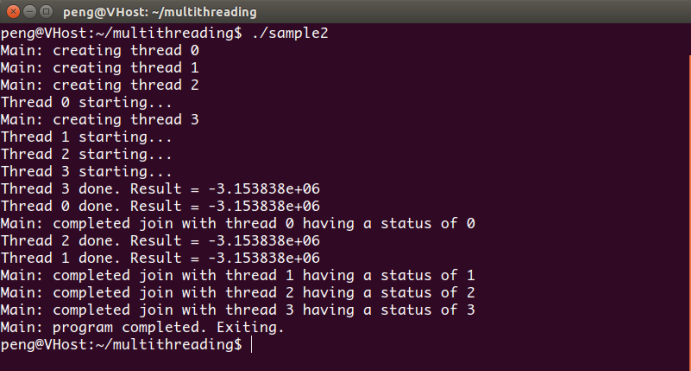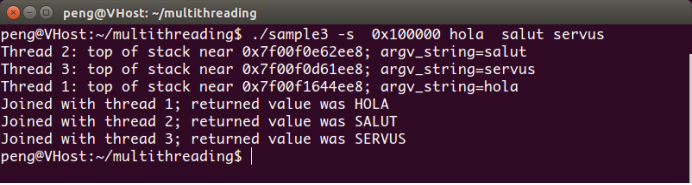1 執行緒 ID

``pthread_t pthread_self(void);``

``int pthread_equal(pthread_t t1, pthread_t t2);``

2 建立與終止

2.1. 建立執行緒

``````int pthread_create(pthread_t *thread, const pthread_attr_t *attr,
void *(*start_routine) (void *), void *arg);``````

 錯誤碼 出錯說明 EAGAIN 系統沒有建立執行緒所需要的資源 EINVAL attr引數無效 EPERM 呼叫程式沒有適當的許可權來設定排程策略或attr指定的引數

• attr 用來表示一個封裝了執行緒各種屬性的屬性物件，如果 attr NULL，新執行緒就使用預設的屬性， 下面第4部分將討論執行緒屬性的細節；

• start_routine 是執行緒開始執行的時候呼叫的函式的名字， start_routine 函式有一個有指向 void 的指標引數，並有 pthread_create 的第四個引數 arg 指定值，同時start_routine 函式返回一個指向 void 的指標，這個返回值被 pthread_join 當做退出狀態處理， 下面第3部分介紹執行緒的退出狀態；

• arg 為引數 start_routine 指定函式的引數。

2.2 終止執行緒

``void pthread_exit(void *retval);``

2.3 執行緒範例-1
``````執行緒的建立與終止 ：
#include <stdio.h>
#include <stdlib.h>

void *PrintHello(void *threadid){ /* 執行緒函式 */
long tid;
printf("Hello World! It's me, thread #%ld!\n", tid); /* 列印執行緒對應的引數 */
}

int main (int argc, char *argv[])
{
int rc;
long t;
for(t=0; t<NUM_THREADS; t++){ /* 迴圈建立 5 個執行緒 */
printf("In main: creating thread %ld\n", t);
if (rc){
printf("ERROR; return code from pthread_create() is %d\n", rc);
exit(-1);
}
}
printf("In main: exit!\n");
return 0;
}
``````3 連線與分離

• 分離執行緒是指執行緒退出時執行緒將釋放它的資源的執行緒；

3.1 執行緒分離

``int pthread_detach(pthread_t thread);``

``pthread_detach(pthread_self());``

3.2 執行緒連線

``int pthread_join(pthread_t thread, void **retval);``

``````pthread_join 函式示例
#include <stdio.h>
#include <stdlib.h>
#include <math.h>

void *BusyWork(void *t) /* 執行緒函式 */
{
int i;
long tid;
double result=0.0;
tid = (long)t;

for (i=0; i<1000000; i++)
{
result = result + sin(i) * tan(i); /* 進行數學運算 */
}
printf("Thread %ld done. Result = %e\n",tid, result);
}

int main (int argc, char *argv[])
{
int rc;
long t;
void *status;

{
if (rc)
{
printf("ERROR; return code from pthread_create() is %d\n", rc);
exit(-1);
}
}

{
if (rc)
{
printf("ERROR; return code from pthread_join() is %d\n", rc);
exit(-1);
}
printf("Main: completed join with thread %ld having a status of %ld\n",t,(long)status);
}

printf("Main: program completed. Exiting.\n");
}``````4 執行緒屬性

4.1 屬性物件

1）初始化屬性物件
``int pthread_attr_init(pthread_attr_t *attr);``

``int pthread_attr_destroy(pthread_attr_t *attr);``

4.2 執行緒狀態

1）獲取執行緒狀態

``int pthread_attr_getdetachstate(pthread_attr_t *attr, int *detachstate);``

2）設定執行緒狀態

``int pthread_attr_setdetachstate(pthread_attr_t *attr, int detachstate);``

4.3 執行緒棧

1）獲取執行緒棧

``int pthread_attr_getstacksize(pthread_attr_t *attr, size_t *stacksize);``

``int pthread_attr_setstacksize(pthread_attr_t *attr, size_t stacksize);``

4.4 執行緒範例-3

``````執行緒屬性示例
#include <string.h>
#include <stdio.h>
#include <stdlib.h>
#include <unistd.h>
#include <errno.h>
#include <ctype.h>

#define handle_error_en(en, msg) \ /* 出錯處理巨集供返回錯誤碼的函式使用 */
do { errno = en; perror(msg); exit(EXIT_FAILURE); } while (0)
#define handle_error(msg) \ /* 出錯處理巨集 */
do { perror(msg); exit(EXIT_FAILURE); } while (0)

char *argv_string;
};

static void *thread_start(void *arg){ /* 執行緒執行函式 */
char *uargv, *p;
printf("Thread %d: top of stack near %p; argv_string=%s\n", /* 通過 p 的地址來計算棧的起始地址*/

uargv = strdup(tinfo->argv_string);
if (uargv == NULL)
handle_error("strdup");
for (p = uargv; *p != '\0'; p++)
*p = toupper(*p); /* 小寫字元轉換大寫字元 */

return uargv; /* 將轉換結果返回 */
}

int main(int argc, char *argv[])
{
int stack_size;
oid *res;
stack_size = -1;

while ((opt = getopt(argc, argv, "s:")) != -1) { /* 處理引數-s 所指定的棧大小 */
switch (opt) {
case 's':
stack_size = strtoul(optarg, NULL, 0);
break;
default:
fprintf(stderr, "Usage: %s [-s stack-size] arg...\n",argv);
exit(EXIT_FAILURE);
}
}

s = pthread_attr_init(&attr); /* 初始化屬性物件 */
if (s != 0)

if (stack_size > 0)
{
s = pthread_attr_setstacksize(&attr, stack_size); /* 設定屬性物件的棧大小 */
if (s != 0)
}

if (tinfo == NULL)
handle_error("calloc");

for (tnum = 0; tnum < num_threads; tnum++)
{
tinfo[tnum].argv_string = argv[optind + tnum];
if (s != 0)
}

s = pthread_attr_destroy(&attr); /* 銷燬屬性物件 */
if (s != 0)

for (tnum = 0; tnum < num_threads; tnum++)
{
if (s != 0)